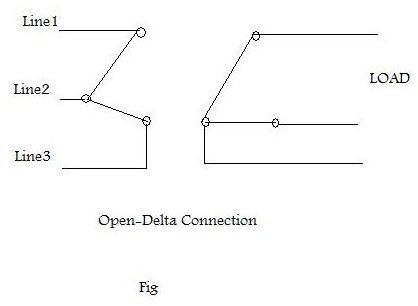# Can an Open-Delta Connection Provide True Three Phase with Two Transformers?

## Open-Delta Connection

In single phase power provision, peak power appears once (for each positive half cycle) in a complete cycle. This is not enough when it comes to driving a motor that produces heavy torque or driving an air conditioner. To overcome the need for heavy power, the three-phase system of power was introduced. In three-phase, peak power appears three times for each half cycle of a complete waveform.

As in single phase, we need a transformer to step-up or step-down 3-phase voltage according to our requirement. For three phase power systems, we have T/Fs having three sets of primary and secondary windings. These two sets of primary and secondary windings can be connected in either star or delta configurations. Both these configurations have their own plus points. Delta configuration provides full load even if one winding is open (failed). Star configuration provides different sets of voltages. So we have four different types of configurations.

1. Delta-Delta: Widely used in industries.
2. Delta-Star: Used in industry and commercial applications.
3. Star-Delta: For transmission purposes.
4. Star-Star: Not commonly used.

In Delta-Delta configuration if we remove one transformer as shown in the figure below (please click to enlarge), we get a configuration called Open–Delta or a V-V connection. This type of configuration is adopted when the power requirement is relatively low and is suitable for small businesses. The resultant output of two T/Fs is truly 3-phase with less relative power than three T/F configurations.## Power Factor with Two Transformers in 3-Phase Open-Delta Configuration

The power factor of a typical T/F is 0.866, so the max output (KVA) of a T/F will be 86.6% of its rated capacity. Suppose we have three transformers each having the same rating (50 KVA). Then the total capacity is equal to 150 * 0.866=130 KVA and not 150 KVA. (The factor 0.866 is called the Utility Factor.)

If one transformer is removed, the total output of two T/Fs will be 100 * 0.866=86.6 KVA. From this calculation, we see that output capacity is reduced when a Delta-Delta configuration is operated as an Open-Delta connection, i.e. with one T/F removed.

Let say the two T/Fs of Open-Delta configuration are supplying 3-phase power to a load having power factor Cosα. The power factor of one T/F is Cos(30-α) and the other is Cos(30+α).

Power (T/F1)=KVA Cos(30-α) and Power(T/F2)= KVACos(30+α)

• Case (i): When phase angle (α=0) is zero, mean load p.f=1 – each transformer has power factor = Cos30 = 0.866
• Case (ii):When phase angle is 30(α=30), mean load p.f=0.866, one T/F will have p.f of cos(30-30) = Cos0 = 1 and the other will be operating at p.f of Cos(30+30) = Cos60 = 0.866
• Case(iii):When phase angle is 60 (α=60), mean load p.f=0.5, then one T/F will have p.f of Cos(30-60) = 0.866 and other will have a p.f of Cos(30+60) = Cos90 = 0.

From the third scenario we conclude that if we have a transformer Open-Delta configuration in which one T/F is transferring power to an entire load at a p.f of 0.866, then the other one will not provide any load.

Applications of Open-Delta configurations

• Widely used for small 3-phase loads.
• Used in applications where the current load is less, and may increase in future.
• There is no relative shift in output, so can be used for unbalanced loads and where harmonics are involved.

References:

Author's own experience

Electrical Technology By A.K Theraja and B.L Theraja.

Electric Machinery Fundamentals by Stephen J.Chapman 3rd Ed.